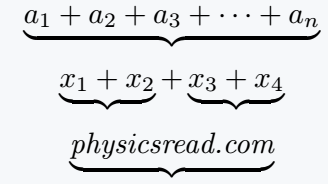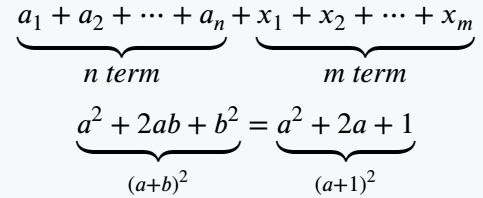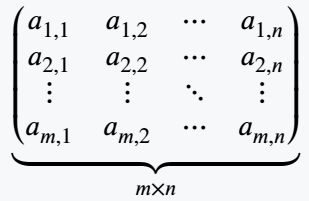# How to write underbrace symbol in LaTeX?

Underbrace is a single curly bracket that is used below the expression. For exampleLatex has many methods to represent this symbol. And in all cases, there are structural differences between the symbols.

This tutorial will cover all the methods so that you can find the solution to all the questions in your mind in this tutorial.

Properties Value
Symbol Underbrace
Argument Yes
Command \underbrace
Example \underbrace{abcd}First, the \underbrace command in latex is defined without any packages. That is, you can use directly this command to represent this symbol. For example

\documentclass{article}
\begin{document}
$$\underbrace{a_1+a_2+a_3+ \cdots + a_n}$$
$$\underbrace{x_1+x_2}+\underbrace{x_3+x_4}$$
$$\underbrace{\textit{physicsread.com}}$$
\end{document}

Output :## Use multiple methods for underbrace symbol in LaTeX

Here multiple packages have been used to explain multiple methods. And each package contains the same command \underbrace but has structural differences, as shown in the table below.

Package Command
MnSymbol \underbrace{abcd}fdsymbol \underbrace{abcd}stix \underbrace{abcd}mathtools \underbrace{abcd}mathabx \underbrace{abcd}abraces \underbrace{abcd}However, the \aunderbrace command has been used for the abraces package.

## Multiple lines underbrace expression in LaTeX

You may have noticed that different text, number is written under the underbrace. And to represent this syntax, you need to use a subscript with this command.

\documentclass{article}
\usepackage{stix}
\begin{document}
$$\underbrace{a_1+a_2+\cdots+a_n}_{\textit{n term}}+\underbrace{x_1+x_2+\cdots+x_m}_{\textit{m term}}$$
$$\underbrace{a^2+2ab+b^2}_{(a+b)^2}=\underbrace{a^2+2a+1}_{(a+1)^2}$$
\end{document}

Output :And the expression is passed as an argument in the subscript to display the expression below the underbrace symbol.

## Use underbrace below matrix in LaTeX

To use a curly bracket under a matrix, the entire matrix environment must be passed within that \underbrace command as an argument.

\documentclass{article}
\usepackage{stix,amsmath}
\begin{document}
$$\underbrace{ \begin{pmatrix} a_{1,1} & a_{1,2} & \cdots & a_{1,n} \\ a_{2,1} & a_{2,2} & \cdots & a_{2,n} \\ \vdots & \vdots & \ddots & \vdots \\ a_{m,1} & a_{m,2} & \cdots & a_{m,n} \end{pmatrix}}_{m \times n}$$
\end{document}

Output :#### Md Jidan Mondal

LaTeX expert with over 10 years of experience in document preparation and typesetting. Specializes in creating professional documents, reports, and presentations using LaTeX.